# Propositional logic facts for kids

Kids Encyclopedia Facts

Propositional logic is a formal system in mathematics and logic. Other names for the system are propositional calculus and sentential calculus. The system is made of a set of propositions. Each proposition has a truth value, being either true or false. Propositions can be represented by capital roman letters such as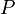$P$,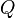$Q$ and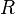$R$, and joined together using logical connectives to make new propositions. Examples for logical connectives that are used often are logical and (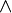$\land$), logical or (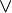$\lor$), logical if (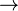$\rightarrow$), logical if and only if (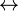$\leftrightarrow$) and logical not ($\lnot$).

Propositional logic only looks at the propositions and how they are connected, and does not decompose them. That way, the proposition All cats are dogs and the earth is a disc is made of two propositions, All cats are dogs, and The Earth is a disc. These are joined together with the logical connective AND.

There are other logic systems that build on propositional logic. One of these is predicate logic, which defines logical predicates, and looks at how they can be applied to arguments. Another system is called modal logic. It introduces two new junctors: it is possible that and it is necessary that.

## If-then statements

A simple logic statement is an implication (statement of the form "If P, then Q"). For example, "If it rains for an hour, then the ground will be wet". In this example, P ("if it rains for an hour") is the antecedent, and Q ("the ground will be wet") is the consequent. The consequent must necessarily follow if the antecedent is true. Therefore, an if-then statement is wholly false if the consequent does not actually follow the antecedent. An example of this would be, "If a dog ate a whole meal, then it would be starving." In this case if P were true, Q would be false and the whole statement is false. Here is a truth table for an if-then statement:

P Q Total Truth Value
True True True
True False False
False True True
False False True

Note that there are two logic errors that people often make from this:

1) "If Q, then P". In our example, this would mean "If the ground is wet, then it has been raining for an hour". However, the ground could be wet for other reasons, like if snow has melted or a water main has broken.

2) "If not P, then not Q". In our example, this would mean "If it has not been raining for an hour, then the ground is not wet". However, the ground could still be wet for the reasons listed above.

The only correct inference that can be made is "If not Q, then not P". This means, in our example, "If the ground is not wet, then it has not been raining for an hour".

## And/ Or statements

Another simple logic statement is "P or Q". For example, "Either I am in Hong Kong or I am in London."

## Related pagesPropositional logic Facts for Kids. Kiddle Encyclopedia.